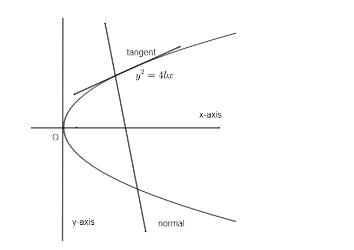Courses
Courses for Kids
Free study material
Free LIVE classes
MoreLIVE
Join Vedantu’s FREE Mastercalss

# If the normal at the point $\left( bt_{1}^{2},2b{{t}_{1}} \right)$ on a parabola, ${{y}^{2}}=4bx$ meets the curve again at point $\left( bt_{2}^{2},2b{{t}_{2}} \right)$ then,(a) ${{t}_{2}}={{t}_{1}}+\dfrac{2}{{{t}_{1}}}$(b) ${{t}_{2}}=-{{t}_{1}}-\dfrac{2}{{{t}_{1}}}$(c) ${{t}_{2}}=-{{t}_{1}}+\dfrac{2}{{{t}_{1}}}$(d) ${{t}_{2}}={{t}_{1}}-\dfrac{2}{{{t}_{1}}}$Verified
335.1k+ views
Hint: o solve this question we will first of all determine the equation of normal of given parabola. The equation of normal of parabola of type, ${{y}^{2}}=4ax$ ar point $\left( {{x}_{1}},{{y}_{1}} \right)$ is given by,
$\left( y-{{y}_{1}} \right)=\dfrac{-1}{\dfrac{dy}{dx}}\left( x-{{x}_{1}} \right)$

Given parabola is, ${{y}^{2}}=4bx$ this parabola and normal would be of the form.We have equation of normal of parabola, ${{y}^{2}}=4ax$ is, $\left( y-{{y}_{1}} \right)=\dfrac{-1}{\left( \dfrac{dy}{dx} \right)}\left( x-{{x}_{1}} \right)$ at point $\left( {{x}_{1}},{{y}_{1}} \right)$ - (1)
Given that equation of parabola is, ${{y}^{2}}=4bx$.
Differentiating both sides with respect to x we get,
\begin{align} & 2y\dfrac{dy}{dx}=4b \\ & \Rightarrow \dfrac{dy}{dx}=\dfrac{4b}{2y} \\ \end{align}
Then, $\dfrac{dy}{dx}=\dfrac{2b}{y}$ - (2)
We are given that the normal is at the point $\left( bt_{1}^{2},2b{{t}_{1}} \right)$.
Substituting value of $y=2b{{t}_{1}}$ in equation (2) we get,
$\Rightarrow \dfrac{dy}{dx}=\dfrac{1\left( 2b \right)}{2b{{t}_{1}}}=\dfrac{1}{{{t}_{1}}}$
Also the slope of normal is $\dfrac{-1}{\left( \dfrac{dy}{dx} \right)}$.
$\Rightarrow$ Slope of normal = $\dfrac{-1}{\left( \dfrac{1}{{{t}_{1}}} \right)}=-{{t}_{1}}$.
Therefore, equation of normal ar $\left( bt_{1}^{2},2b{{t}_{1}} \right)$ is,
$\Rightarrow \left( y-2b{{t}_{1}} \right)=-{{t}_{1}}\left( x-bt_{1}^{2} \right)$ - (3)
Now the point $\left( bt_{2}^{2},2b{{t}_{2}} \right)$ also lies on the normal. Therefore, point $\left( bt_{2}^{2},2b{{t}_{2}} \right)$ satisfies (3) we get,
$\Rightarrow \left( 2b{{t}_{2}}-2b{{t}_{1}} \right)=-{{t}_{1}}\left( bt_{2}^{2}-bt_{1}^{2} \right)$
Taking 2b common on left we get, and also taking b common on right;
$\Rightarrow 2b\left( {{t}_{2}}-{{t}_{1}} \right)=-{{t}_{1}}b\left( t_{2}^{2}-t_{1}^{2} \right)$
Now applying identity $\left( {{a}_{2}}-{{a}_{1}} \right)\left( {{a}_{2}}+{{a}_{1}} \right)=a_{2}^{2}-a_{1}^{2}$ on the RHS of above equation we get,
$\Rightarrow 2b\left( {{t}_{2}}-{{t}_{1}} \right)=-{{t}_{1}}b\left( {{t}_{2}}-{{t}_{1}} \right)\left( {{t}_{2}}+{{t}_{1}} \right)$
Now cancelling $b\left( {{t}_{2}}-{{t}_{1}} \right)$ on both sides we get,
This can be done as $b\ne 0$ & ${{t}_{2}}-{{t}_{1}}\ne 0$.
\begin{align} & \Rightarrow 2=-{{t}_{1}}\left( {{t}_{2}}+{{t}_{1}} \right) \\ & \Rightarrow -{{t}_{1}}\left( {{t}_{2}}+{{t}_{1}} \right)=2 \\ & \Rightarrow -{{t}_{2}}{{t}_{1}}=2+t_{1}^{2} \\ \end{align}
Dividing by ${{t}_{1}}$ we get,
$\Rightarrow -{{t}_{2}}=\dfrac{2+t_{1}^{2}}{{{t}_{1}}}$
Multiplying ‘minus’ both sides we get,
$\Rightarrow {{t}_{2}}=-\dfrac{2}{{{t}_{1}}}-{{t}_{1}}$
$\Rightarrow {{t}_{2}}=-{{t}_{1}}-\dfrac{2}{{{t}_{1}}}$, which is option (b).
So, the correct answer is “Option B”.

Note: The possibility of error in this question can be at a point where students directly substitute value of point $\left( bt_{2}^{2},2b{{t}_{2}} \right)$ in equation of parabola. This would be wrong because this point $\left( bt_{2}^{2},2b{{t}_{2}} \right)$ is a point of contact normal of parabola. So, we first need to determine the parabola normal of parabola then we can proceed accordingly.
Last updated date: 26th Sep 2023
Total views: 335.1k
Views today: 4.35k